Precalculus

# Chapter 6

PrecalculusChapter 6

### 6.1Graphs of the Sine and Cosine Functions

1.

$6π 6π$

2.

$1 2 1 2$ compressed

3.

$π 2 ; π 2 ;$ right

4.

2 units up

5.

midline: $y=0; y=0;$ amplitude: $| A |= 1 2 ; | A |= 1 2 ;$ period: $P= 2π | B | =6π; P= 2π | B | =6π;$ phase shift: $C B =π C B =π$

6.

$f( x )=sin(x)+2 f( x )=sin(x)+2$

7.

two possibilities: $y=4sin( π 5 x− π 5 )+4 y=4sin( π 5 x− π 5 )+4$ or $y=−4sin( π 5 x+ 4π 5 )+4 y=−4sin( π 5 x+ 4π 5 )+4$

8.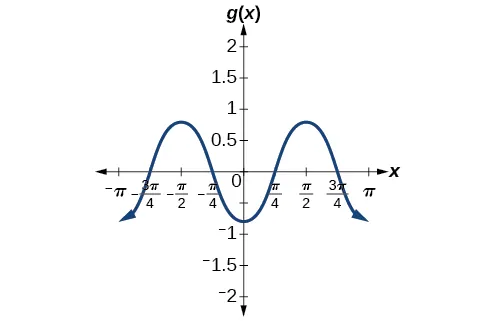midline: $y=0; y=0;$ amplitude: $| A |=0.8; | A |=0.8;$ period: $P= 2π | B | =π; P= 2π | B | =π;$ phase shift: $C B =0 C B =0$ or none

9.midline: $y=0; y=0;$ amplitude: $| A |=2; | A |=2;$ period: $P= 2π | B | =6; P= 2π | B | =6;$ phase shift: $C B =− 1 2 C B =− 1 2$

10.

711.

$y=3cos( x )−4 y=3cos( x )−4$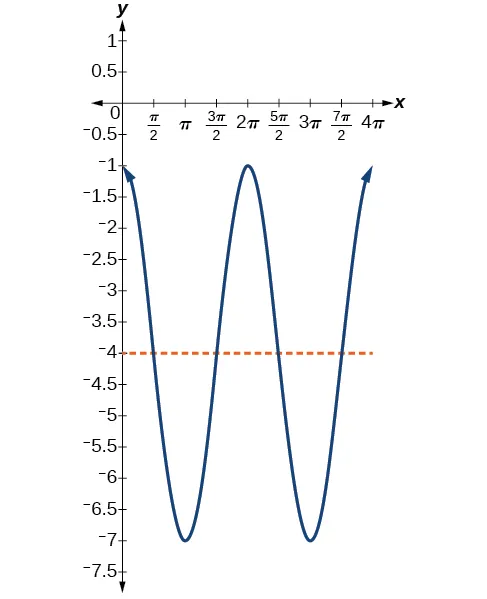### 6.2Graphs of the Other Trigonometric Functions

1.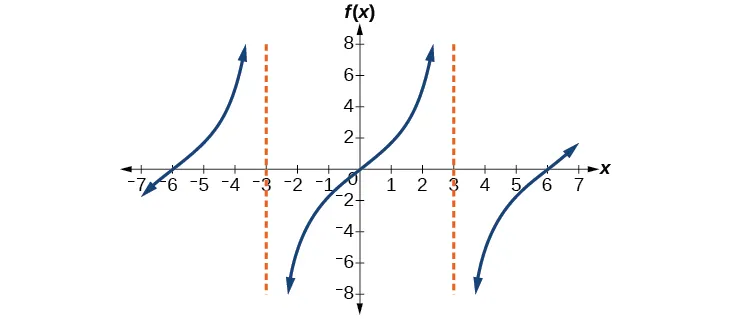2.

It would be reflected across the line $y=−1, y=−1,$ becoming an increasing function.

3.

$g(x)=4tan(2x) g(x)=4tan(2x)$

4.

This is a vertical reflection of the preceding graph because $A A$ is negative.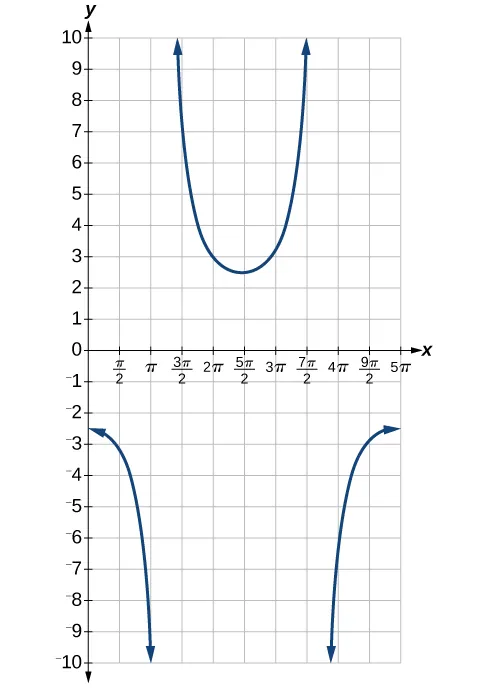5.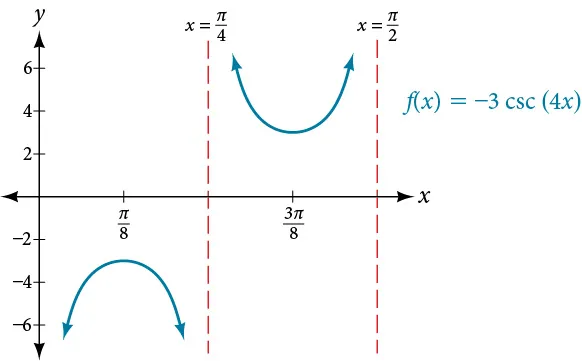6.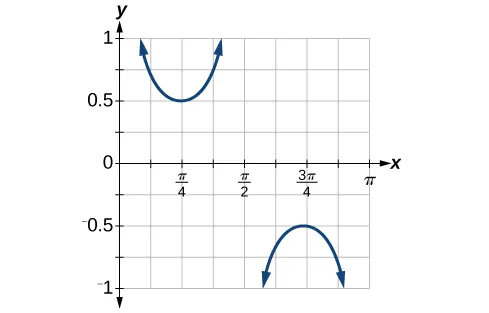7.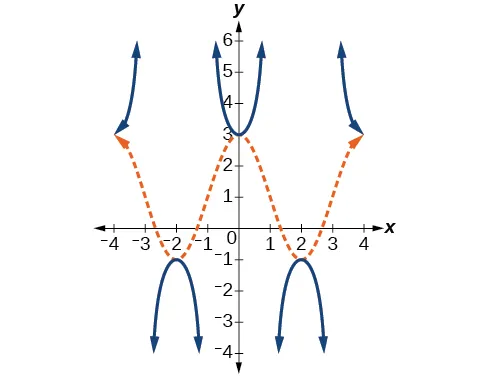### 6.3Inverse Trigonometric Functions

1.

$arccos(0.8776)≈0.5 arccos(0.8776)≈0.5$

2.
1. $− π 2 ; − π 2 ;$
2. $− π 4 ; − π 4 ;$
3. $π; π;$
4. $π 3 π 3$
3.

1.9823 or 113.578°

4.

$sin −1 (0.6)=36.87°=0.6435 sin −1 (0.6)=36.87°=0.6435$ radians

5.

$π 8 ; 2π 9 π 8 ; 2π 9$

6.

$3π 4 3π 4$

7.

$12 13 12 13$

8.

$4 2 9 4 2 9$

9.

$4x 16 x 2 +1 4x 16 x 2 +1$

### 6.1 Section Exercises

1.

The sine and cosine functions have the property that $f( x+P )=f( x ) f( x+P )=f( x )$ for a certain $P. P.$ This means that the function values repeat for every $P P$ units on the x-axis.

3.

The absolute value of the constant $A A$ (amplitude) increases the total range and the constant $D D$ (vertical shift) shifts the graph vertically.

5.

At the point where the terminal side of $t t$ intersects the unit circle, you can determine that the $sint sint$ equals the y-coordinate of the point.

7.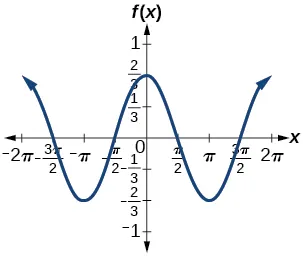amplitude: $2 3 ; 2 3 ;$ period: $2π; 2π;$ midline: $y=0; y=0;$ maximum: $y= 2 3 y= 2 3$ occurs at $x=0; x=0;$ minimum: $y=− 2 3 y=− 2 3$ occurs at $x=π; x=π;$ for one period, the graph starts at 0 and ends at $2π 2π$

9.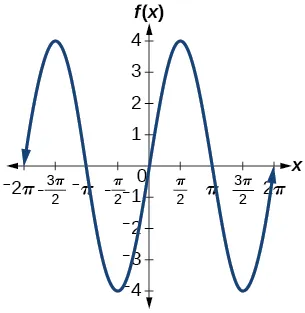amplitude: 4; period: $2π; 2π;$ midline: $y=0; y=0;$ maximum $y=4 y=4$ occurs at $x= π 2 ; x= π 2 ;$ minimum: $y=−4 y=−4$ occurs at $x= 3π 2 ; x= 3π 2 ;$ one full period occurs from $x=0 x=0$ to $x=2π x=2π$

11.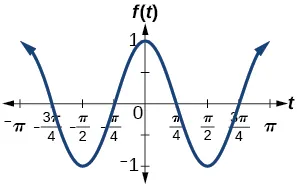amplitude: 1; period: $π; π;$ midline: $y=0; y=0;$ maximum: $y=1 y=1$ occurs at $x=π; x=π;$ minimum: $y=−1 y=−1$ occurs at $x= π 2 ; x= π 2 ;$ one full period is graphed from $x=0 x=0$ to $x=π x=π$

13.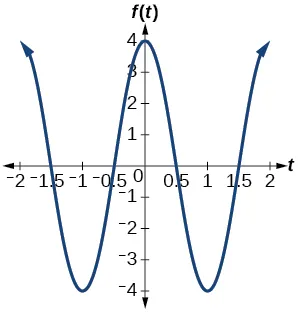amplitude: 4; period: 2; midline: $y=0; y=0;$ maximum: $y=4 y=4$ occurs at $x=0; x=0;$ minimum: $y=−4 y=−4$ occurs at $x=1 x=1$

15.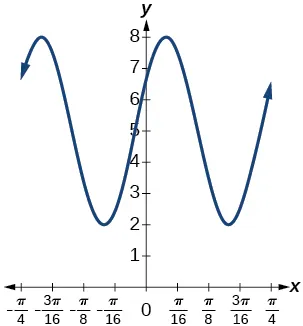amplitude: 3; period: $π 4 ; π 4 ;$ midline: $y=5; y=5;$ maximum: $y=8 y=8$ occurs at $x=0.12; x=0.12;$ minimum: $y=2 y=2$ occurs at $x=0.516; x=0.516;$ horizontal shift: $−4; −4;$ vertical translation 5; one period occurs from $x=0 x=0$ to $x= π 4 x= π 4$

17.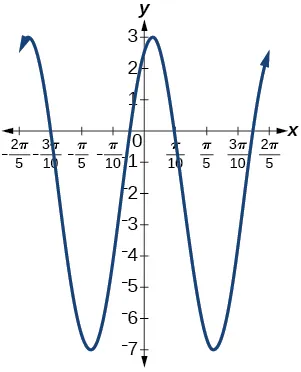amplitude: 5; period: $2π 5 ; 2π 5 ;$ midline: $y=−2; y=−2;$ maximum: $y=3 y=3$ occurs at $x=0.08; x=0.08;$ minimum: $y=−7 y=−7$ occurs at $x=0.71; x=0.71;$ phase shift: $−4; −4;$ vertical translation: $−2; −2;$ one full period can be graphed on $x=0 x=0$ to $x= 2π 5 x= 2π 5$

19.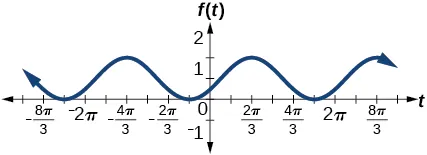amplitude: 1 ; period: $2π; 2π;$ midline: $y=1; y=1;$ maximum: $y=2 y=2$ occurs at $x=2.09; x=2.09;$ maximum: $y=2 y=2$ occurs at $t=2.09; t=2.09;$ minimum: $y=0 y=0$ occurs at $t=5.24; t=5.24;$ phase shift: $− π 3 ; − π 3 ;$ vertical translation: 1; one full period is from $t=0 t=0$ to $t=2π t=2π$

21.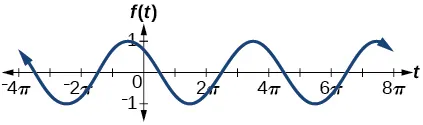amplitude: 1; period: $4π; 4π;$ midline: $y=0; y=0;$ maximum: $y=1 y=1$ occurs at $t=11.52; t=11.52;$ minimum: $y=−1 y=−1$ occurs at $t=5.24; t=5.24;$ phase shift: $− 10π 3 ; − 10π 3 ;$ vertical shift: 0

23.

amplitude: 2; midline: $y=−3; y=−3;$ period: 4; equation: $f(x)=2sin( π 2 x )−3 f(x)=2sin( π 2 x )−3$

25.

amplitude: 2; period: 5; midline: $y=3; y=3;$ equation: $f(x)=−2cos( 2π 5 x )+3 f(x)=−2cos( 2π 5 x )+3$

27.

amplitude: 4; period: 2; midline: $y=0; y=0;$ equation: $f(x)=−4cos( π( x− π 2 ) ) f(x)=−4cos( π( x− π 2 ) )$

29.

amplitude: 2; period: 2; midline $y=1; y=1;$ equation: $f( x )=2cos( πx )+1 f( x )=2cos( πx )+1$

31.

$0,π 0,π$

33.

$sin(π2)=1 sin(π2)=1$

35.

$π2 π2$

37.

$f(x)=sinx f(x)=sinx$ is symmetric

39.

$π3,5π3 π3,5π3$

41.

Maximum: $1 1$ at $x= 0 x=0$ ; minimum: $-1 -1$ at $x= π x=π$

43.

A linear function is added to a periodic sine function. The graph does not have an amplitude because as the linear function increases without bound the combined function $h(x)=x+sinx h(x)=x+sinx$ will increase without bound as well. The graph is bounded between the graphs of $y=x+1 y=x+1$ and $y=x-1 y=x-1$ because sine oscillates between −1 and 1.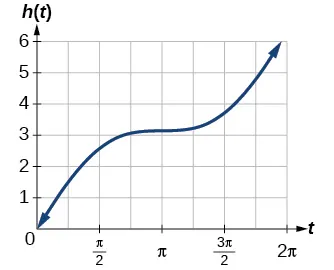45.

There is no amplitude because the function is not bounded.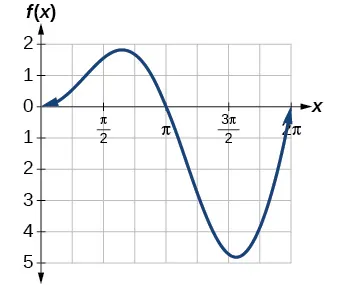47.

The graph is symmetric with respect to the y-axis and there is no amplitude because the function’s bounds decrease as $|x| |x|$ grows. There appears to be a horizontal asymptote at $y=0 y=0$ .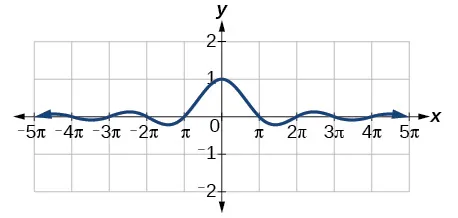### 6.2 Section Exercises

1.

Since $y=cscx y=cscx$ is the reciprocal function of $y=sinx, y=sinx,$ you can plot the reciprocal of the coordinates on the graph of $y=sinx y=sinx$ to obtain the y-coordinates of $y=cscx. y=cscx.$ The x-intercepts of the graph $y=sinx y=sinx$ are the vertical asymptotes for the graph of $y=cscx. y=cscx.$

3.

Answers will vary. Using the unit circle, one can show that $tan( x+π )=tanx. tan( x+π )=tanx.$

5.

The period is the same: $2π. 2π.$

7.

IV

9.

III

11.

period: 8; horizontal shift: 1 unit to left

13.

1.5

15.

5

17.

$−cotxcosx−sinx −cotxcosx−sinx$

19.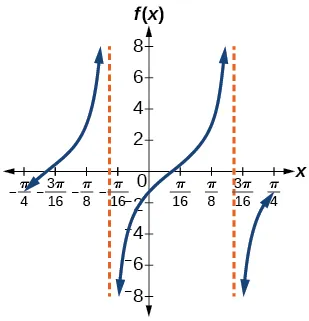stretching factor: 2; period: $π 4 ; π 4 ;$ asymptotes:

21.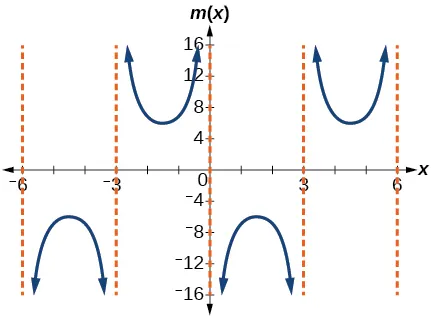stretching factor: 6; period: 6; asymptotes:

23.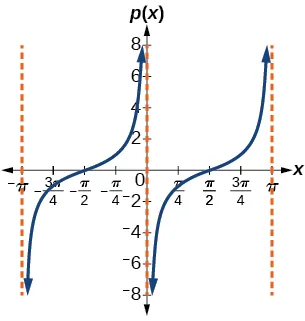stretching factor: 1; period: $π; π;$ asymptotes:

25.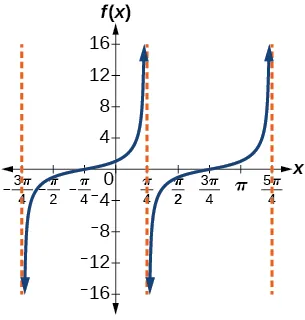Stretching factor: 1; period: $π; π;$ asymptotes:

27.stretching factor: 2; period: $2π; 2π;$ asymptotes:

29.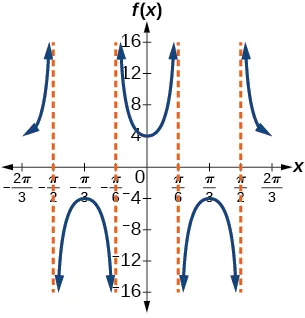stretching factor: 4; period: $2π 3 ; 2π 3 ;$ asymptotes:

31.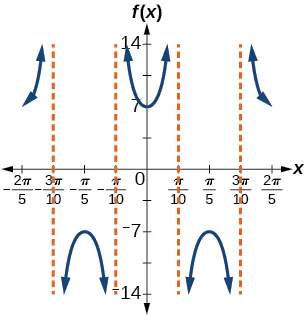stretching factor: 7; period: $2π 5 ; 2π 5 ;$ asymptotes:

33.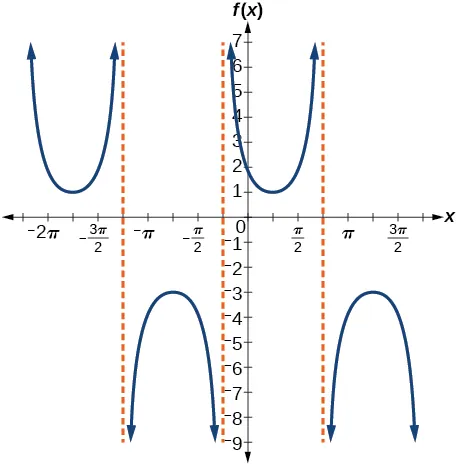stretching factor: 2; period: $2π; 2π;$ asymptotes:

35.stretching factor: $7 5 ; 7 5 ;$ period: $2π; 2π;$ asymptotes:

37.

$y=tan( 3( x− π 4 ) )+2 y=tan( 3( x− π 4 ) )+2$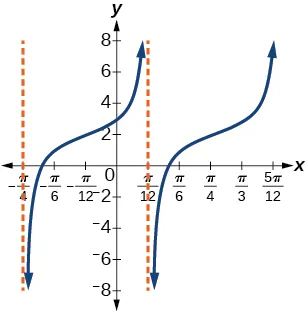39.

$f( x )=csc( 2x ) f( x )=csc( 2x )$

41.

$f( x )=csc( 4x ) f( x )=csc( 4x )$

43.

$f( x )=2cscx f( x )=2cscx$

45.

$f(x)= 1 2 tan(100πx) f(x)= 1 2 tan(100πx)$

47.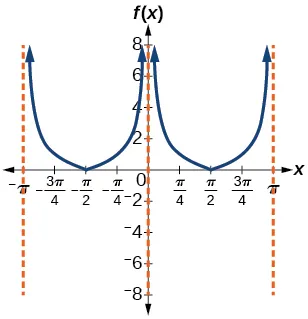49.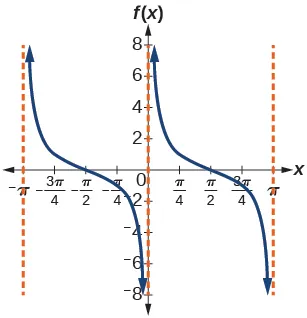51.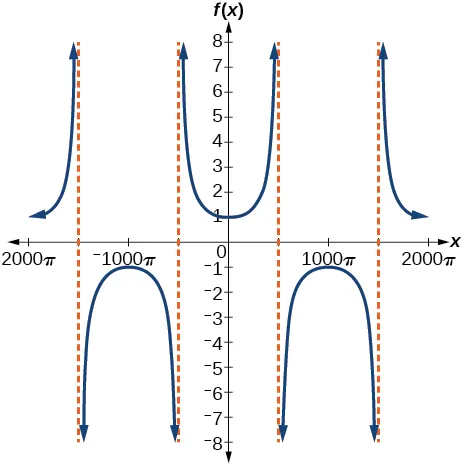53.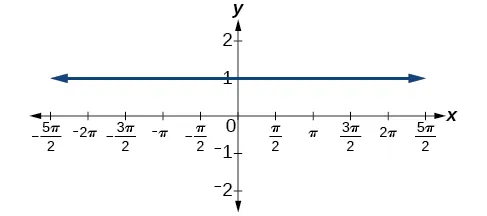55.
1. $( − π 2 , π 2 ); ( − π 2 , π 2 );$
2.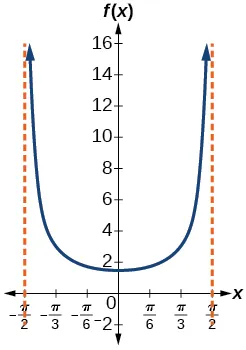3. $x=− π 2 x=− π 2$ and $x= π 2 ; x= π 2 ;$ the distance grows without bound as $| x | | x |$ approaches $π 2 π 2$ —i.e., at right angles to the line representing due north, the boat would be so far away, the fisherman could not see it;
4. 3; when $x=− π 3 , x=− π 3 ,$ the boat is 3 km away;
5. 1.73; when $x= π 6 , x= π 6 ,$ the boat is about 1.73 km away;
6. 1.5 km; when $x=0 x=0$
57.
1. $h( x )=2tan( π 120 x ); h( x )=2tan( π 120 x );$
2.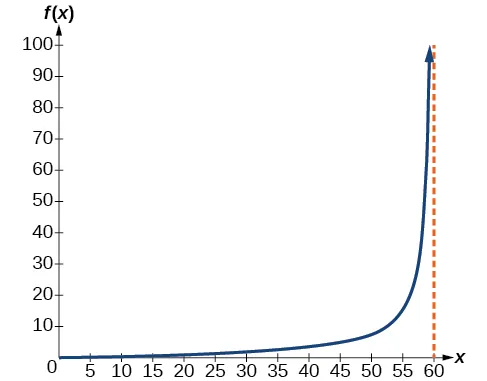3. $h( 0 )=0: h( 0 )=0:$ after 0 seconds, the rocket is 0 mi above the ground; $h( 30 )=2: h( 30 )=2:$ after 30 seconds, the rockets is 2 mi high;
4. As $x x$ approaches 60 seconds, the values of $h( x ) h( x )$ grow increasingly large. The distance to the rocket is growing so large that the camera can no longer track it.

### 6.3 Section Exercises

1.

The function $y=sinx y=sinx$ is one-to-one on $[ − π 2 , π 2 ]; [ − π 2 , π 2 ];$ thus, this interval is the range of the inverse function of $y=sinx, y=sinx,$ $f(x)= sin −1 x. f(x)= sin −1 x.$ The function $y=cosx y=cosx$ is one-to-one on $[ 0,π ]; [ 0,π ];$ thus, this interval is the range of the inverse function of $y=cosx,f(x)= cos −1 x. y=cosx,f(x)= cos −1 x.$

3.

$π 6 π 6$ is the radian measure of an angle between $− π 2 − π 2$ and $π 2 π 2$ whose sine is 0.5.

5.

In order for any function to have an inverse, the function must be one-to-one and must pass the horizontal line test. The regular sine function is not one-to-one unless its domain is restricted in some way. Mathematicians have agreed to restrict the sine function to the interval $[ − π 2 , π 2 ] [ − π 2 , π 2 ]$ so that it is one-to-one and possesses an inverse.

7.

True . The angle, $θ 1 θ 1$ that equals $arccos(−x) arccos(−x)$, $x>0 x>0$, will be a second quadrant angle with reference angle, $θ 2 θ 2$, where $θ 2 θ 2$ equals $arccosx arccosx$, $x>0 x>0$. Since $θ 2 θ 2$ is the reference angle for $θ 1 θ 1$, $θ 2 =π− θ 1 θ 2 =π− θ 1$ and $arccos(−x) arccos(−x)$ = $π−arccosx π−arccosx$-

9.

$− π 6 − π 6$

11.

$3π 4 3π 4$

13.

$− π 3 − π 3$

15.

$π 3 π 3$

17.

1.98

19.

0.93

21.

1.41

23.

25.

0

27.

0.71

29.

-0.71

31.

$− π 4 − π 4$

33.

0.8

35.

$5 13 5 13$

37.

$x−1 − x 2 +2x x−1 − x 2 +2x$

39.

$x 2 −1 x x 2 −1 x$

41.

$x+0.5 − x 2 −x+ 3 4 x+0.5 − x 2 −x+ 3 4$

43.

$2x+1 x+1 2x+1 x+1$

45.

$2x+1 x 2x+1 x$

47.

$t t$

49.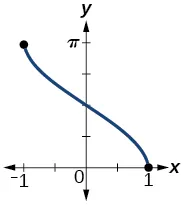domain $[ −1,1 ]; [ −1,1 ];$ range $[ 0,π ] [ 0,π ]$

51.

approximately $x=0.00 x=0.00$

53.

55.

57.

59.

61.

No. The angle the ladder makes with the horizontal is 60 degrees.

### Review Exercises

1.

amplitude: 3; period: $2π; 2π;$ midline: $y=3; y=3;$ no asymptotes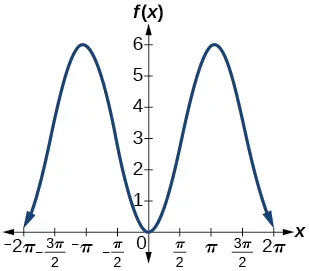3.

amplitude: 3; period: $2π; 2π;$ midline: $y=0; y=0;$ no asymptotes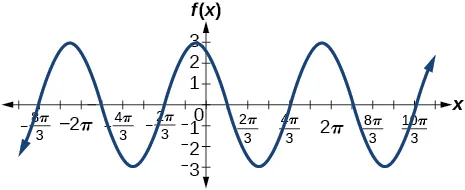5.

amplitude: 3; period: $2π; 2π;$ midline: $y=−4; y=−4;$ no asymptotes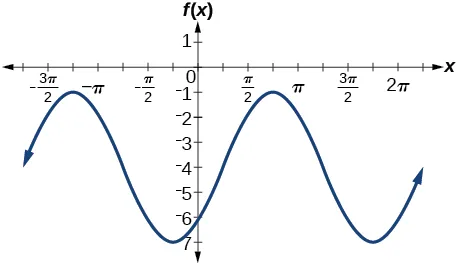7.

amplitude: 6; period: $2π 3 ; 2π 3 ;$ midline: $y=−1; y=−1;$ no asymptotes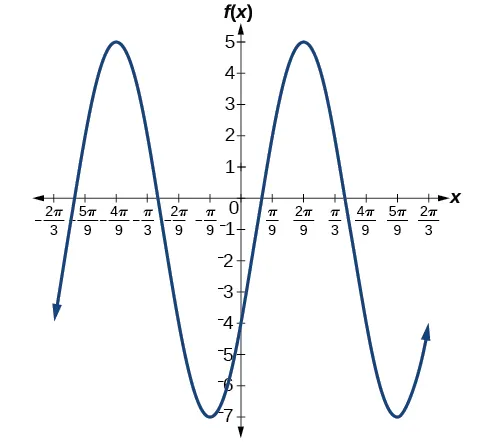9.

stretching factor: none; period: $π; π;$ midline: $y=−4; y=−4;$ asymptotes: $x= π 2 +πk, x= π 2 +πk,$ where $k k$ is an integer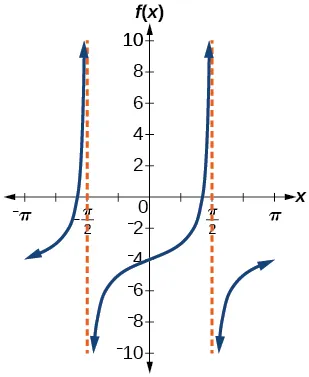11.

stretching factor: 3; period: $π 4 ; π 4 ;$ midline: $y=−2; y=−2;$ asymptotes: $x= π 8 + π 4 k, x= π 8 + π 4 k,$ where $k k$ is an integer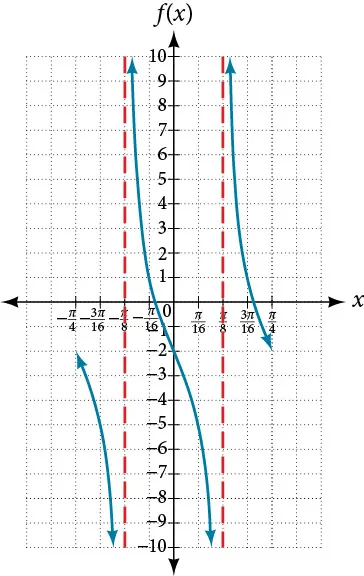13.

amplitude: none; period: $2π; 2π;$ no phase shift; asymptotes: $x= π 2 k, x= π 2 k,$ where $k k$ is an odd integer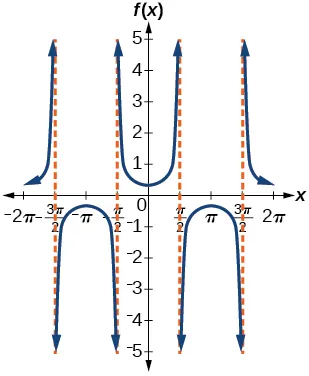15.

amplitude: none; period: $2π 5 ; 2π 5 ;$ no phase shift; asymptotes: $x= π 5 k, x= π 5 k,$ where $k k$ is an integer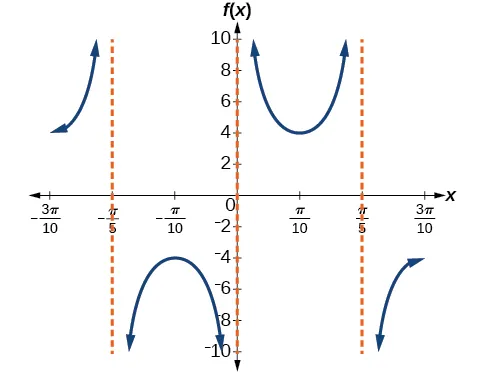17.

amplitude: none; period: $4π; 4π;$ no phase shift; asymptotes: $x=2πk, x=2πk,$ where $k k$ is an integer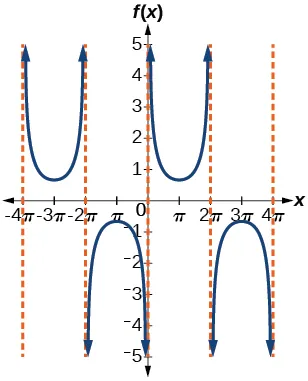19.

largest: 20,000; smallest: 4,000

21.

amplitude: 8,000; period: 10; phase shift: 0

23.

In 2007, the predicted population is 4,413. In 2010, the population will be 11,924.

25.

5 in.

27.

10 seconds

29.

$π 6 π 6$

31.

$π 4 π 4$

33.

$π 3 π 3$

35.

No solution

37.

$12 5 12 5$

39.

The graphs are not symmetrical with respect to the line $y=x. y=x.$ They are symmetrical with respect to the $y y$-axis.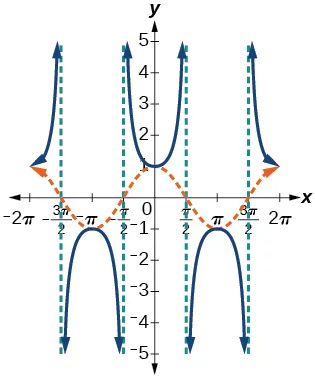41.

The graphs appear to be identical.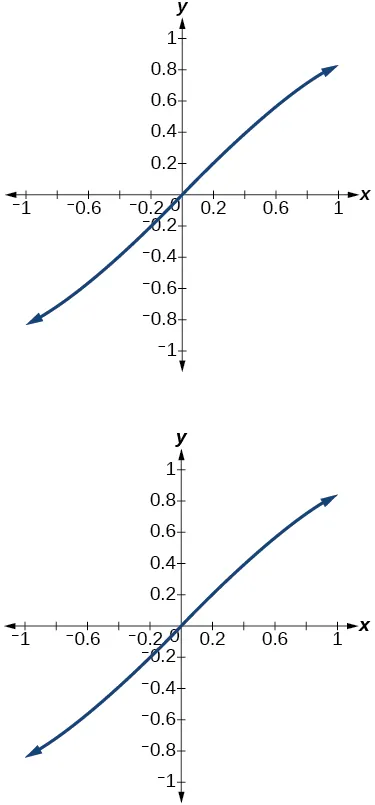### Practice Test

1.

amplitude: 0.5; period: $2π; 2π;$ midline $y=0 y=0$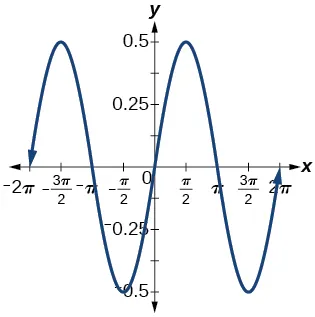3.

amplitude: 5; period: $2π; 2π;$ midline: $y=0 y=0$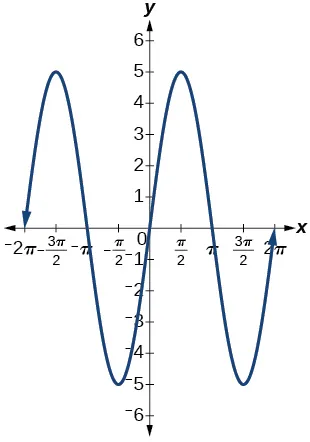5.

amplitude: 1; period: $2π; 2π;$ midline: $y=1 y=1$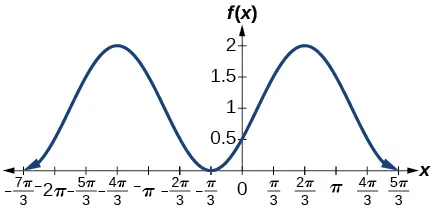7.

amplitude: 3; period: $6π; 6π;$ midline: $y=0 y=0$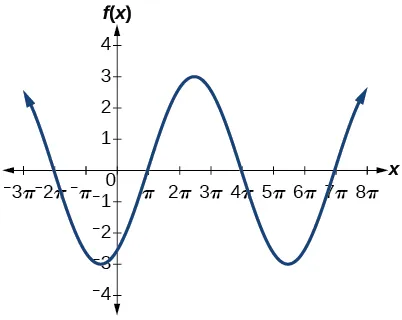9.

amplitude: none; period: $π; π;$ midline: $y=0, y=0,$ asymptotes: $x= 2π 3 +πk, x= 2π 3 +πk,$ where $k k$ is an integer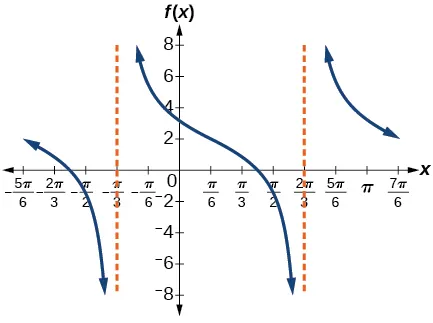11.

amplitude: none; period: $2π 3 ; 2π 3 ;$ midline: $y=0, y=0,$ asymptotes: $x= π 3 k, x= π 3 k,$ where $k k$ is an integer13.

amplitude: none; period: $2π; 2π;$ midline: $y=−3 y=−3$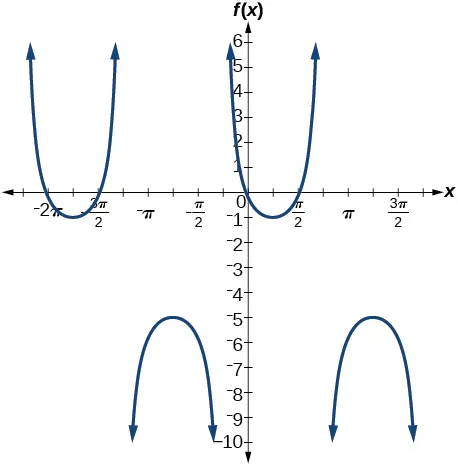15.

amplitude: 2; period: 2; midline: $y=0; y=0;$ $f( x )=2sin( π( x−1 ) ) f( x )=2sin( π( x−1 ) )$

17.

amplitude: 1; period: 12; phase shift: $−6; −6;$ midline $y=−3 y=−3$

19.

$D( t )=68−12sin( π 12 x ) D( t )=68−12sin( π 12 x )$

21.

period: $π 6 ; π 6 ;$ horizontal shift: $−7 −7$

23.

$f( x )=sec( πx ); f( x )=sec( πx );$ period: 2; phase shift: 0

25.

$4 4$

27.

The views are different because the period of the wave is $1 25 . 1 25 .$ Over a bigger domain, there will be more cycles of the graph.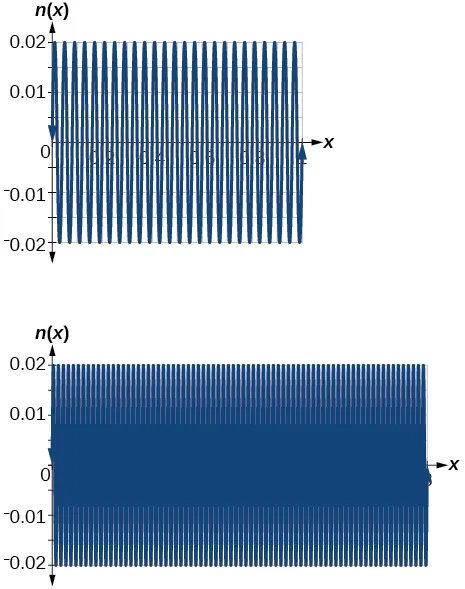29.

$3 5 3 5$

31.

On the approximate intervals $( 0.5,1 ),( 1.6,2.1 ),( 2.6,3.1 ),( 3.7,4.2 ),( 4.7,5.2 ),(5.6,6.28) ( 0.5,1 ),( 1.6,2.1 ),( 2.6,3.1 ),( 3.7,4.2 ),( 4.7,5.2 ),(5.6,6.28)$

33.

$f( x )=2cos( 12( x+ π 4 ) )+3 f( x )=2cos( 12( x+ π 4 ) )+3$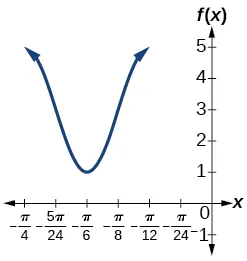35.

This graph is periodic with a period of $2π. 2π.$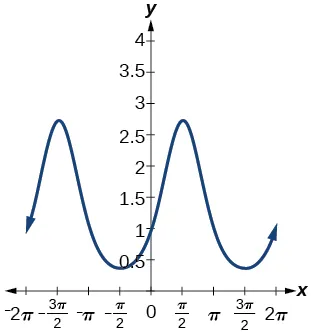37.

$π 3 π 3$

39.

$π 2 π 2$

41.

$1− ( 1−2x ) 2 1− ( 1−2x ) 2$

43.

$1 1+ x 4 1 1+ x 4$

45.

$x+1 x x+1 x$

47.

False

49.

Order a print copy

As an Amazon Associate we earn from qualifying purchases.